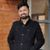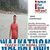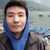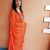# About Marginal cost of capital

Why is the marginal cost of capital the relevant concept for evaluating investment projects, rather than a firm’s actual historic cost of capital?

This post was part of TyroCity discussion forumAngel Paudel

The marginal cost of capital in simple terms can be referred to as additional cost incurred from the additional unit of capital risen (Taggart, 1991). Clarifying this with an example, when we’re borrowing money, people with better credit score and lesser risk will get lower interest rate while the rest with have slightly higher as per their risk level. Same is true for a company. Consider that they borrowed the most they could and soon they run out of the credit line from the bank. They have another option in taking out a credit card or other investors, the business in debt now has a higher risk and thus the interest rate is higher as well. So, as you keep borrowing more and more and more, the cost of capital is going to be a higher cost of capital as well. For example, with the company have 0 borrowing can borrow upto \$1m at 4% interest rate but the rate will increase once that point is crossed, let’s say to 6% for the next \$0.5m and it keeps on rising as the risk level, leverage of the company ability to pay off debt is lesser as well.

Historical cost specifically refers to the value of the assets that are to be found in the balance sheet. It records the original or purchased price of the asset and is shown without any changes in the historical cost (Otley, 1999). Even though they don’t include any personal opinions about how much should an asset be valued in the current time, it’s far less accurate. Let’s see this with an example. A company purchased a computer at \$1,000 in 2016, the cost now of the used laptop would be somewhere around \$400 however, it’s still recorded to be of \$1,000 itself. Another example of the value on rise but being recorded the same would in the case of a building. Let’s assume that the company buys its corporate building at a cost of \$1m in 2000. The value rose over the period tenfold every ten years and is now around \$100m in 2018. However, the cost is recorded to be no different, which isn’t practical.

The marginal cost of capital makes the comparisons of assets and analysis on its changing value possible which can’t be done with the historical cost. Also, Marginal cost gives a business idea about the current aspect of the market and current valuations rather than the past record which isn’t practical in the current age. In the current world, where we can see the price of petroleum products changing every 15-days in Nepal and real-time pricing implemented in India and other parts of the world, we can’t say that the price of any asset will remain the same even after years of it being purchased by a company like in the case of historical price if used. Marginal cost is thus more accurate, gives a real picture, is reliable and assist in the decision-making process is why its relevant concept of evaluating a firm’s investment projects rather than going with the historical cost of capital.

References

Otley, D. (1999). Performance management: a framework for management control systems research. Management Accounting Research , 10 (4), 363-365.

Taggart, R. (1991). Consistent Valuation and Cost of Capital Expressions with Corporate and Personal Taxes. Financial Management , 20 (3), 8.Cost of Capital:

First, here I am going to discuss on cost of capital. A firm’s cost of capital is the rate the firm has to pay for the use of debt, preferred stock, common stock, and/or retained earnings. In other words it is the rate that must be earned on the firm’s investment in order to satisfy all the investors required rate of return. Cost of capital is an important concept in financial decision making. It is used as standard for evaluating investment projects, designing capital structure and determining appropriate discounting rate to compute net present value (NPV) of cash flow and to compare with internal rate of return (IRR) for evaluating worthiness of investment proposals (Michael, 2016).

A firm’s should compute component cost of capital to determine weighted average cost of capital. Before - tax cost of debt, kd, is the rate of return required by the debt holders. After - tax cost of debt, kdt, is computed by multiplying before - tax cost of debt (1 - tax rate). Cost of preferred stock, kps, is the rate of return required by the preferred stockholders. Similarly, a firm’s cost of equity capital is defined as the rate of return required by its common stock holders. Equity capital can be raised internally through retained earnings and externally through the scale of new common stock. Cost of internal equity, ks, can be computed using dividend discounting model, capital assert pricing model, and using bond - yield - plus risk premium approach. Cost of external equity, ke, is greater than the cost of internal equity because of issuances expanses.

Marginal Cost:

The marginal cost of capital is weighted average cost of capital of last rupees of new financing. It is also called weighted marginal cost of capital (WMCC). The value minimizing firm user the WMCC which is based on target capital structure to evaluate the investment proposal. The break-even point helps to calculate WMCC (Arnold, 2008). The level of financing after which the WACC shifts upward is called break point.

Historic Cost of Capital Vs. Marginal Cost of Capital:

In economics marginal cost of any item is the cost of another unit of that item. For example, marginal cost of output is the cost of adding one additional unit of output. The same concept applies to cost of capital. The marginal cost of capital is the cost of raising an additional rupees of capital. But in practice, the capital is raised in lump sum. Hence, the marginal cost of capital in finance refers to the cost incurred in raising the incremental funds (Maness, 2009). Thus, the weighted average cost of new or incremental capital is known as the marginal cost of capital.

We computed Sunrise Company’s weighted average cost of capital to be 10.6 percent. As long as sunrise keeps its capital structure constant, as long as its component costs versus cost of debt after - tax (6%), cost of preferred stock (12%) and cost of equity (14%), then it WACC will be 10.6%. This means each rupees the company raised will consist of some long-term debt, some preferred stock, and some common equity, and the cost of the whole rupees will be 10.6 % its marginal cost of capital (MACC) will be 10.6%.

Marginal cost of capital does not remain constant. As the volume of financing increase the costs of various sources of financing increase. Because suppliers of funds required greater returns in form of interest, dividend, or growth as compensation for the increased risk introduced by large volumes of new financing, other reasons of higher cost for large amount of financing are flotation costs, underpricing of the security, discount etc, Marginal cost of capital therefore an increasing function of level new financing.

References
Arnold, G. (2008). Corporate financial management. , FT Prentice Hall, Pearson Education .

Maness, S. (2009). Introduction to Corporate Finance. McGraw-Hill, New York, USA.

Michael, T. J. (2016). Do You Know Your Cost of Capital? Harvard Business Review .Marginal Cost

"Though there may be differences as to its exact method of calculation, there is general agreement that the marginal cost of capital is an important criterion when making investment decisions. An integral part of this computation is the company’s retained earnings. If the objective of the marginal cost of capital is to serve as a criterion for the investment decision, the computed marginal cost of capital should reflect its intended value. (Hollinger, 1978)” Marginal cost of capital is the cost incurred in producing one additional unit of product or service. This is calculated as

Marginal cost = change in costs/change in quantity

The change is cost is calculated by deducting the total cost with cost incurred before production of additional unit. Similarly, the change in quantity is calculated as total quantity minus the previous quantity before additional quantity. This cost calculates the cost related with one additional unit and is used to evaluate how much production will make the companies cost of producing the lowest considering the economies of scale.

Marginal cost usually calculated the operating cost of production of a unit as the fixed asset is already there and will not incur cost. Therefore to get the actual marginal cost, calculation are done for a longer period so fixed assets are also a part of marginal cost.

It is calculated as

WACC = (wd)(kd)(1-t) + (wps)(kps) + (wce)(kce)

The WACC calculates the average cost of capital by taking the weight of all the variables involved.

Historic cost of capital

It is also known as the net cash outlay (NCO). When a company invests in a project or service, it invests through either debt or equity. The return from investment is expected to be higher than the NCO. Thus, the required rate of return forms a benchmark to be met by the investment. This takes into consideration of the risk factor. Higher the risk higher the expected rate of return. ‘Cost of Capital’ is relevant while structuring the capital requirements of a company, for deciding the right mix of means of financing and for assessing the success or otherwise of any project. It forms the basis of return any investment has to earn so as to maximize shareholder wealth. (Gopalan, 2011)

This cost of capital is a projection for future. Therefore the data taken from past history is only relevant for project to be done from inside the company. So for projects outside the company, the cost of capital generated from history of the company will not work.

A company’s investment generally includes both debt and equity so it is necessary to calculate both the cost of debt and cost of equity to find the cost of capital. The cost of debt from past history may not be relevant for future forecasting as the rate and type of debt may vary in the future.

It is calculated as

Cost of capital = Cost of debt + Cost of equity

Cost of debt (kd) = C/Po

Where, C = Coupon Amount and Po = Current Market price

Cost of Equity (Re) = Rm + Rf * B

Where Rm = Market risk premium, Rf = Risk free rate and B = beta risk

Thus marginal cost calculates the cost of additional cost of production which is a better method to calculate investing in securities rather than cost of capital that takes historic data which may not be relevant in future.

References

Gopalan, V. (2011, March). Relevance of ‘Cost of Capital’ in investment decision making. Management Accountant; Calcutta , 217-218.

Hollinger, R. D. (1978, April). The marginal cost of capital and retained earnings. Financial Executive; New York , p. 56.Cost of capital refers to the discount rate used for calculating the present value of the future cash flow streams (Moyer, McGuigan, Rao, & Kretlow, 2012). Historical cost of capital refers to cost of the fund that has already been used for financing a project. The historic cost of capital is calculated based on the past data. The weight of each component capital used in a project with their respective cost is used in determining historic coat of capital. It helps in evaluating the past performance of the firm by comparing it with the standard costs of capital and predicting the future costs (Classification of Cost of Capital).

Marginal cost of capital (MCC) can be defined as the cost of raising an additional rupee of capital. It is the cost incurred in raising new funds. It is derived by calculating average cost of capital using marginal weights, the proportion of the funds the firm intends to invest. The marginal cost increases with the increase in capital raised due to the flotation cost involved in the process of issuing sticks for generating additional capital. However, it may be equal to the average cost of capital if the funds are raised in proportional manner and the component cost of capital remain unchanged.

The most significant role of marginal cost of capital is to evaluate the new investment project. If the rate of return offered by the project is equal to greater than the MCC, it is selected otherwise we reject it. The marginal cost is relevant for evaluating new investment project because of the following reasons;

• It eliminates the confusion regarding whether to use book value weight or market value weight of capital in computing cost of capital.

• It provides real time cost of capital for the proposed project that helps in taking more objective decision.

• It reflects current opportunity cost available for the firm along with the current risk associated with the investment project.

• It helps in calculating the break-even point: the point where marginal rate of return earned on new investment will equal the MCC.

• The concept of historical cost of capital and marginal cost of capital can be clarified with the help of following example:

• A company is using 40% debt and 60% equity to finance a project with respective cost of each component being 15% and 12%. The weighted average cost of capital will be 0.415+0.612 = 13.2%.

• If the company decides to raise new capital by issuing common stock that requires floatation coat of 2% and will change the capital structure to 30% debt and 70% equity. Then the MCC of the firm will be 0.315+0.7(12+2) = 14.3%.

Thus the marginal cost of capital increases with the increase in amount of capital raised.

References
Classification of Cost of Capital. (n.d.). Retrieved from MBA Knowledge Base: mbaknol.com/financial-management/c...

Moyer, R. C., McGuigan, J. R., Rao, R., & Kretlow, W. J. (2012). Contemporary Financial Management (12th ed.). Oklahoma: Cengage Learning.